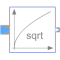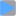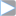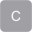# Sqrt

Output the square root of the input (= principal square root of complex number)# Information

This information is part of the Modelica Standard Library maintained by the Modelica Association.

This blocks computes the output `y` as square root of the input `u` (= principal square root of the complex input). Optionally, the input `u` can be processed conjugate complex, when parameter `useConjugateInput` is `true`. Depending on `useConjugateInput` the internal signal `uInternal` represents either the original or the conjugate complex input signal.

```    y = sqrt(uInternal);
```

# Parameters (1)

useConjugateInput Value: false Type: Boolean Description: If true, input is processed conjugate complex

# Connectors (2)

u yType: ComplexInput Description: Connector of Complex input signalType: ComplexOutput Description: Connector of Complex output signal

# Components (1)

 uInternalType: Complex Description: Equals either u or conjugate complex input u if useComplexInput = true